# Form Builder: Calculated Fields

Calculated Fields allow you to mark particular fields with values, and then calculate these values together in a particular way.  They are most commonly used within a scoring rubric for a private review portal.  An example would be, a review must score an applicant's (a) academic success; (b) athletic success; and (c) financial need, when evaluating that applicant for a particular scholarship.  A calculated field could add together the score given to each of these three categories in order to provide us with an overall, holistic score (a+b+c = total score).

### Prepare for a Calculated Field

A Calculated Field is an available field type in the form builder, in the Speciality Fields section.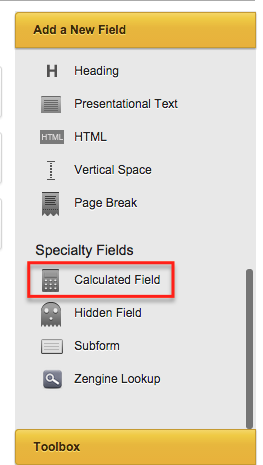However, please note that a Calculated Field will not be usable until you have added all fields to your form that will be added to your calculation and then saved the form.

### Basic Calculated Field

If we continue to use the academics/athletics/financials example from above, then let's look at our first step in creating a Calculated Field.  Here, we have added the three fields which will ultimately be used in our total score.  We opted to utilize dropdowns in order to restrict a reviewer to a finite set of options; a radio button would accomplish the same.  One line text boxes are not advisable if you want to restrict your reviewers to a particular set of numbers/values.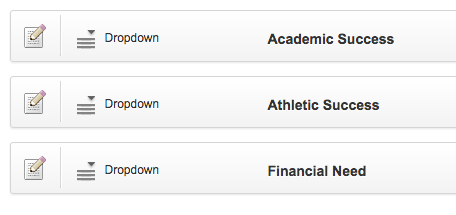Imagining that a review could assign a value from 1-5 for each of these categories. In the choices area, you would put:
1
2
3
4
5

One value per line, without any commas, etc.

Once all items to be pulled into the calculated field are added to your form, and the options for each of these fields have the choices set, we are ready to create a calculation around those values.  You can save your form.

### Create a Calculated Field

When your form is saved, you can now drag and drop a calculated field into your form builder, below the fields which will be used in the calculation.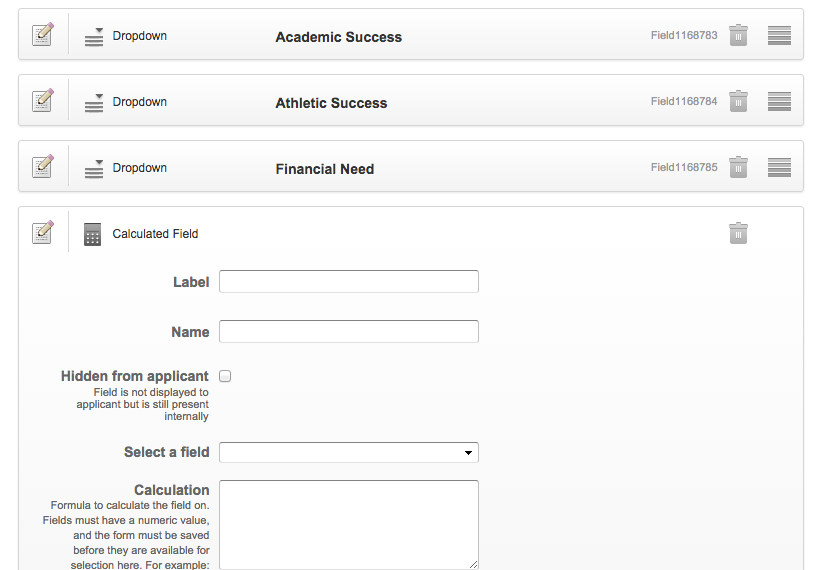You will see there are two special settings: the Select a Field dropdown and the Calculation textbox.

Select a Field will contain all one-line text boxes, dropdowns, checkboxes, and radio buttons on your form.  You should locate the field(s) used in your calculation. By clicking them, the field number associated with that field will automatically appear in the Calculation textbox.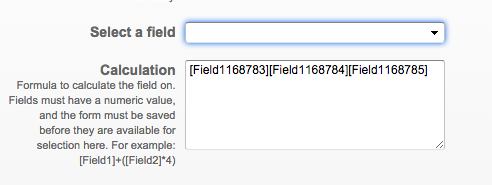Here, we have clicked Academic Success, Athletic Success, and Financial Need.  The field number for each has appeared within brackets.  Now it's time to set up the calculation.

In this simpler example, we just want the three values to be added together to give us a total score out of a possible total 15 points.  So, we just need to add plus signs [+] between each of our values, like so: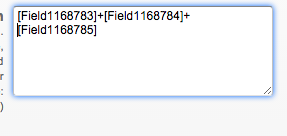If we were to save the form, we now have a calculated field that will automatically add up a reviewer's responses as he/she completes each field.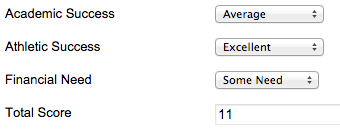### Calculated Fields with Values

Let's imagine that you'd prefer reviewers review according to a criteria other than numeric values; for example, Poor, Below Average, Average, Good, and Excellent instead of 1, 2, 3, 4, and 5 - but that you also want to calculate the reviews with the system recognizing that Excellent is best and Poor is worst.  We can assign hidden values to any selection in a dropdown or selection box.
If we look at Academic Success, we will see that each item has a number, a colon, and a phrase.  The number represents the value, or how much that particular selection is worth.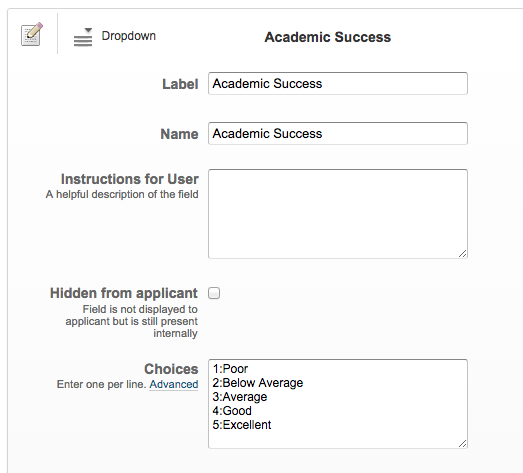Here, Poor is worth 1 point, while Excellent is worth 5 points.  The review will not see the values assigned to their selections.  Anything before the colon is hidden from the reviewer (or applicant) and can be used in a calculated field later on.

### Weighted Values

In the example above, each selection was worth equal value.  The 5 points for financial aid were the same as the 5 points for academic success.  Let's imagine that the most important for our scholarship is academic success, and we want it to be worth three times as much as the other two items.  We can give weight to any values in our Calculation text box.  For this example, we are going to make Academic Success worth three times as much.  So we will multiply Academic Success by 3.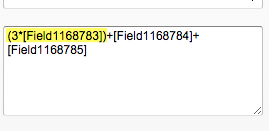Parentheses were created in order to separate this multiplication from the rest of the calculation.  An asterisk [*] was used as the multiple symbol.

### Complex Calculations

To take this a step further, you can really perform any number of calculations within a calculated field. Imagine it is a graphing calculator.  Brackets and parentheses are of utmost importance in order for your field to calculate correctly.  If we had left out the parentheses in the above weighted example, the calculation would have multiplied all 3 values by 3.

Some important symbols to get you started: